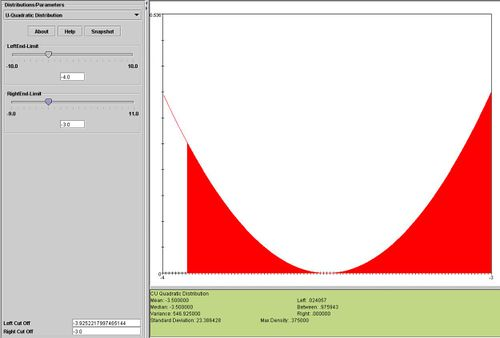### Description

The U quadratic distribution is defined by the following density function

$$f(x)=\alpha \left ( x - \beta \right )^2, \forall x \in [a , b], a < b$$,

where the relation between the two pairs of parameters (domain-support, a and b) and (range/offset $$\alpha$$ and $$\beta$$) are given by the following two equations

(gravitational balance center) $$\beta = {b+a \over 2}$$, and
(vertical scale) $$\alpha = {12 \over \left ( b-a \right )^3}$$.

### Properties

• Support Parameters$a < b \in (-\infty,\infty)$
• Scale/Offset Parameters$\alpha \in (0,\infty)$ and $$\beta \in (-\infty,\infty)$$
• PDF$f(x)=\alpha \left ( x - \beta \right )^2, \forall x \in [a , b]$
• CDF $$F(x)={\alpha \over 3} \left ( (x - \beta)^3 + (\beta - a)^3 \right ), \forall x \in [a , b]$$
• Mean${a+b \over 2}$
• Median${a+b \over 2}$
• Modes$a$ and $$b$$
• Variance${3 \over 20} (b-a)^2$
• Skewness: 0 (distribution is symmetric around the mean)
• Kurtosis${3 \over 112} (b-a)^4$
• Moment Generating Function$M_x(t)= {-3\left(e^{at}(4+(a^2+2a(-2+b)+b^2)t)- e^{bt} (4 + (-4b + (a+b)^2)t)\right) \over (a-b)^3 t^2 }$
• Characteristic Function${3i\left(e^{iat}(-4i+(a^2+2a(-2+b)+b^2)t)+ e^{ibt} (4i - (-4b + (a+b)^2)t)\right) \over (a-b)^3 t^2 }$